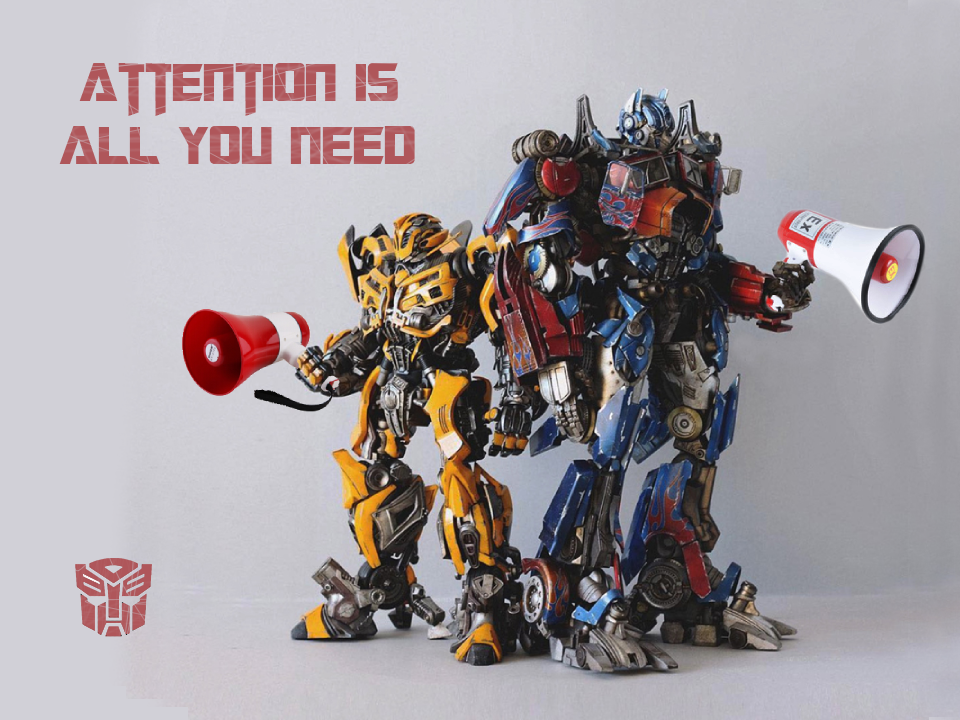## Attention

$\mathrm{Attention}(Q, K, V) = \mathrm{softmax}\left(\frac{QK^\top}{\sqrt{d_k}}\right)V$

$\mathrm{Attention}(q_t, K, V) = \sum\limits_{s=1}^m\frac 1Z\exp\left(\frac{q_t k_s^\top}{\sqrt{d_k}}\right)v_s\qquad t=0, 1, \cdots, n$

\begin{aligned} \mathrm{MultiHead}(Q, K, V) &= \mathrm{Concat}(head_1, head_2, \cdots, head_h)\\ head_i &= \mathrm{Attention} (Q_i,K_i,V_i )\\ Q_i &= QW_i^Q\\ K_i &= KW_i^K\\ V_i &= VW_i^V \end{aligned}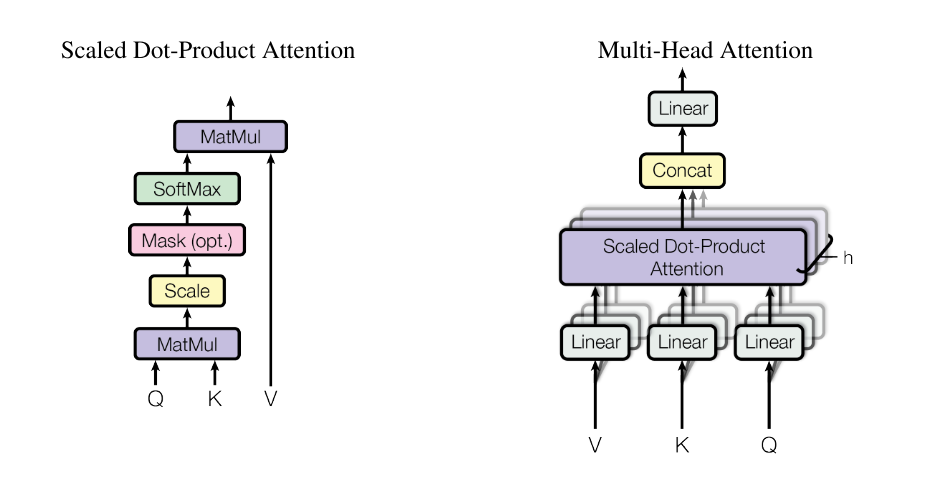## Model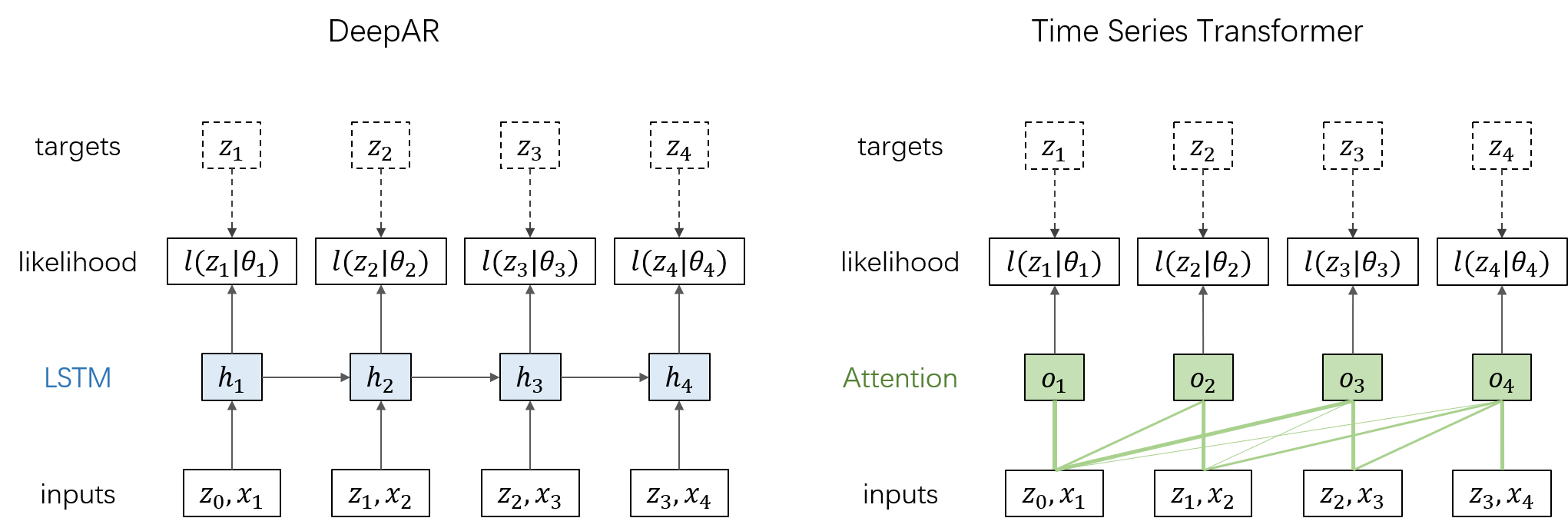$head_i = \mathrm{softmax}\left(\frac{Q_iK_i^\top}{\sqrt{\tilde{d_k}}}\cdot mask\right)V_i\\ Q_i = XW_i^Q,\quad K_i = XW_i^K,\quad V_i = XW_i^V\\$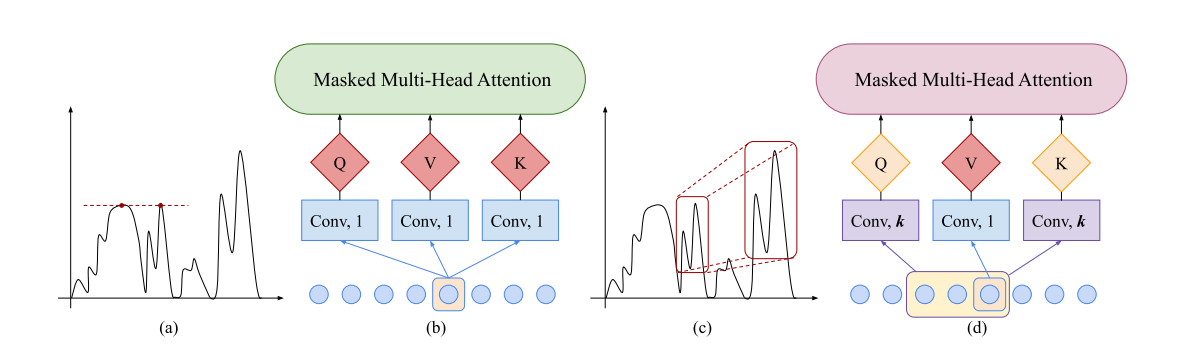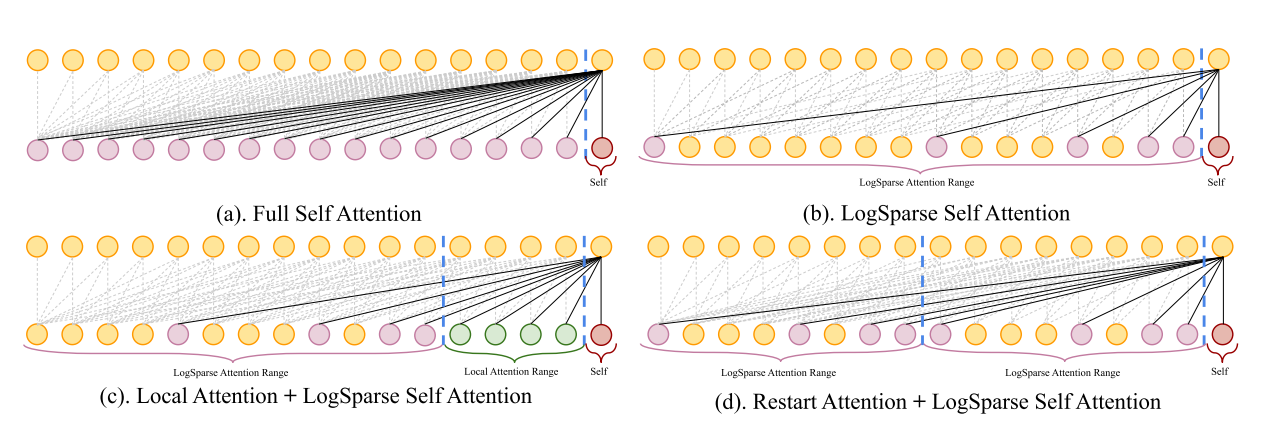## Code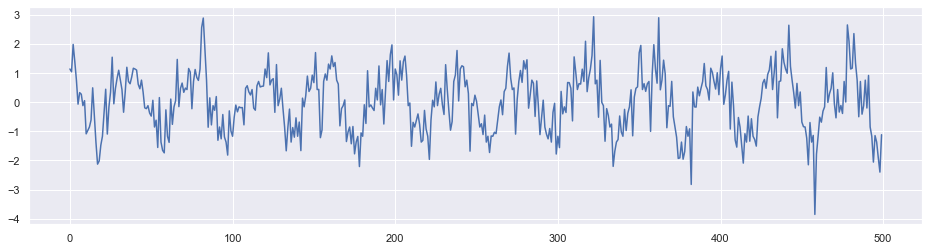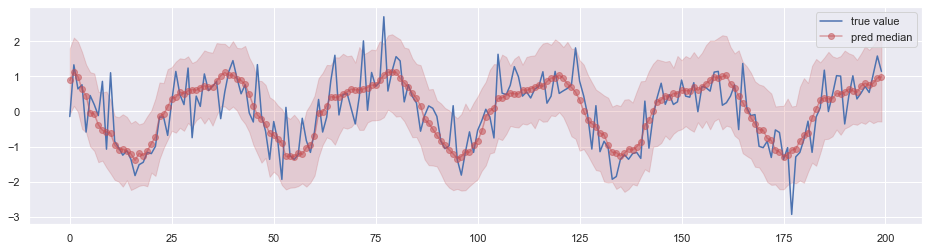## 与 DeepAR 对比

• 从某种意义上来说，两者的网络结构很像，学习的也都是概率分布的参数。
• Attention 结构本身不能很好地捕捉序列的顺序，当然这个不是大问题，因为通常来说时序预测任务都会有时间特征，不需要像自然语言处理时那样加入额外的位置编码。
• 该文章中给出的实验结果表明 Time Series Transformer 在捕捉长程依赖方面比 DeepAR 更有优势。
• Time Series Transformer 在训练的时候可以并行计算，这是优于 DeepAR 的。不过因为和 DeepAR 一样采用了自回归结构，预测的时候无法并行。不仅如此，DeepAR 预测单个时间步时只需要使用当前输入和上一步输出的隐态即可；而 Transformer 却需要计算全局的 Attention。因此在预测的时候，Transformer 的计算效率很可能不如 DeepAR。

1. 严格来说，该文章使用的并不是 Transformer。Google 的 Transformer 是一个 Encoder-Decoder 的结构，而该文章所使用的网络结构实际上是把 DeepAR 中的 LSTM 层替换为 Multi-Head Self Attention 层。这个结构其实一个（不完整的） Transformer Decoder 部分。But, whatever… ↩︎

2. 原文没有这种说法。 ↩︎

3. 这个图是我自己画的，原文没有。 ↩︎